## Recommended Posts

4+5=9

2 + 5 = 7 ?

couldn't help but go with primes

2 + 6 = 8

4+6=10

6+4=10

Good morning!, I wake up just now and I go quickly to forum... well, I see that is not yet the answer correct, but someone has gone very near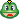4 + 8 = 12?

I have no idea what gritty is? Some type of enamel or something?

I believe that he is referring to the twelve textures that the Mints use. You can specify the texture be put onto a specific area of the coin in order to give it some "depth". The mint will sometime refer to at Grit #1, Grit #2 etc.

Here is a picture of a texture wheel coin

Texture Wheel

Yeah, this is the wheel, thanks scificollector!

4+7=11

Nice coin!!Thanks for the fun!!

6+5=11

I don't even understand the question.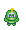But it's a beautiful coin!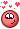4+7=11

Nice coin!!Thanks for the fun!!

I don't even understand the question.But it's a beautiful coin!I'm glad you like!Rules in post #36 (help in post #48)

2 + 8 = 10?

3+8=11

Is it important in which order the figures are, that means is 3+8=11 the same as 8+3=11?

5+6=11

3+8=11

Is it important in which order the figures are, that means is 3+8=11 the same as 8+3=11?

No, is not the same5+3=8 ?

3 + 2 = 5?

5 + 2 = 7

2+5=7

Well i lost that last post since i didn't realize that set was already taken. Oh well gotta wait a couple of hours.

3 + 9 = 12

2 + 9 = 11

6+2=8

4 + 3 = 7

7 x 5 = 12

Thank you!

Aaaaagh! Why did i do that!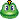7+2=9

8 + 3 = 11

5 + 4 = 9

8 + 2 = 10

4+2=6

7+4=11

10+2=12

5+2=7

1+2=3

Have left a lot of combinations!!, but any is the right answer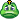, ...in the next cointest (if any) I'll put a spreadsheet to delete the combinations already posted4 + 5 = 9?

Grrr, mistyped....

2+10=12

7 + 5 = 12

Just for clarity!6 + 4 = 106+3=9

7+3=10

8+1=9

2+1=3

11+1=12

1 + 7 = 8

thanks for the cointest,

k

6 + 2 =8

5+5=10

4+4=8

## Join the conversation

You can post now and register later. If you have an account, sign in now to post with your account.
Note: Your post will require moderator approval before it will be visible.×   Pasted as rich text.   Paste as plain text instead

Only 75 emoji are allowed.